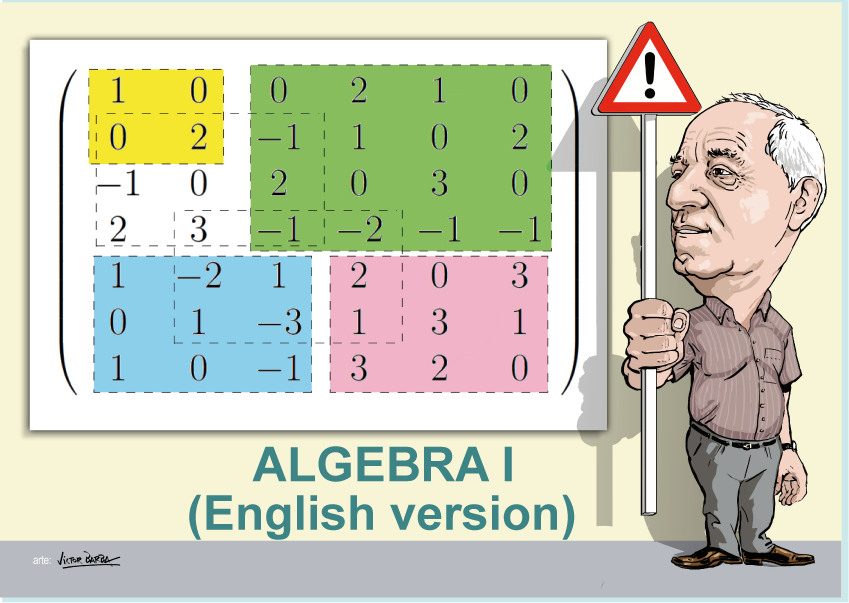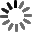El sitio web de la UCLM utiliza cookies propias y de terceros con fines técnicos y de análisis, pero no recaba ni cede datos de carácter personal de los usuarios. Sin embargo, puede haber enlaces a sitios web de terceros, con políticas de cookies distintas a la de la UCLM, que usted podrá aceptar o no cuando acceda a ellos.

Puede obtener más información en la Política de cookies. Aceptar# Algebra I (English)

Curso de fuentes OpenUCLM

The main originality of this course consists in that all discussed problems of linear algebra are solved using a single algorithm, that gives the orthogonal subspace of a linear subspace and its complementary subspace. This permits analyzing all problems from the orthogonality point of view, which is very reach. For example, the problem of determining whether or not a vector belongs to a subspace or the intersection of two subspaces are solved by looking to them as orthogonalization problems. The algorithm permits inverting a matrix, calculating its determinant or determining its rank very easily. In addition the problems of updating inverses and determinants when changing a row reduce to a single step of the algorithm. The compatibility of systems of equations and the obtention of all its solutions or detecting infeasibility are also a direct application of the algorithm. In addition, all subsystems of a given linear system can be solved without extra calculations. Finally, some examples of illustrative applications are given

Autores Fecha Enrique Castillo 06/09/2019 Ingles

Me GustaIn this first block the presentation of the G9 course will be made, exposing the block structure of the same and its sequential planning.

An algebra course based on orthogonality. All algebra problems are solved using an orthogonalization algorithm. It contains examples of engineering applications.
The algebra course I is motivated. One single algorithm, that gives the orthogonal subspace of a linear subspace y presented and used to solve all common problems of linear algebra. The point of view of orthogonality enriches the view of the different problems of linear algebra, including the subspace intersections, linear systems of equations, compatibility of linear systems of equations, etc. Several interesting example of applications are given.

In this first block we will study the orthogonalization algorithm, which although designed to obtain the subspace orthogonal to a given subspace and its complement subspace, will be used later to solve all the linear algebra problems that we will see in this course.

In this lesson we present the orthogonalization algorithm with which all the algebra problems that are going to be solved will be solved. The algorithm receives this name, since it obtains the subspace orthogonal to a given subspace and its complementary subspace. To do this, the algorithm with examples is described step by step.
In this lesson it is shown how the orthogonalization algorithm can be used to obtain the orthogonal subspace of a linear subspace. The properties of theis algorithm are incredible, as it is shown.

Elemental transformations of matrices

Elemental transformations of matrices

In this lesson we explain how to transform matrices using post and pre multiplication by matrices, which produce column and row transformations, respectively. Three types of matrices are defined, which come from the identity matrix replacing one diagonal element, a non-diagonal element or permuting two rows or columns. These matrices are used to produce the same transformations as the algorithm in the different iterations. They are used to demonstrate the formula for the determinant of a matrix.

This second block is focused on the algebraic applications of the algorithm, which include: the calculation of matrix inverses and their updating when changing rows, the calculation of the determinant, the calculation of the rank of a matrix and a base of a linear subspace, the membership of a vector to a linear subspace and the intersection of subspaces.

In this lesson it is explained how the orthogonalization algorithm can be used to obtain the inverse of a matrix. At the same time, the determinant of the matrix is obtained. The pproblem of updating the inverse and the determinant of a matrix after changing a row is deal with. It is demonstrated that a single step allows this updating without the need of repeating the whole process.
In this leson we describe how the orthogonalization algorithm can be used to obtain the rank of a matrix. The method is very simple, because we determine if a row or column vector of the matrix belongs to the subspace of the previous uones and even we obtain the coefficients for the linear combination. The method is much simpler than the one based on obtaining the minors, a large collection of determinants that must be non null to get the rank.
In this lesson we explain how to use the orthogonal algorithm to know if one or more vectors belong to a linear subspace. The method is very simple and fast. It is based on the concept of orthogonality, that is, we use the point of view of orthogonality to solve the problem.
In this lesson we use the orthogonalization algorithm to obtain the intersection of two subspaces. We use the point of view of orthoganilty to realize thet the intersection is the orthogonal subspace to the dual of the first subspace in the second subspace or the orthogonal subspace to the dual of the second subspace in the first subspace

This block focuses on linear systems of equations, including homogeneous and complete systems. It also explains how all the subsystems of a given system can be solved simultaneously, as well as how to analyze the compatibility of a system, that is, whether or not it has a solution.

In this lesson we show how the orthogonal algorithm can be used to solve a linear system of homogeneous equations. It is shown that the solution of a linear system of homogeneous equations is the orthogonal subspace to the linear subspace generated by the row vectors of the coefficients of the system of equations. Thus, a direct application of the algorithm leads to the solution of this problem.
In this lesson we explain how to use the orthogonalization algorithm to solve a complete linear system of equations. First, a virtual unknown is used to transform the system into an homogeneous system plus an additional equation. Later the homogeneous system is solved, and finally, the last equation is imposed. This permits obtaining all solutions as the sum of a linear space plus a particular solution.

Compatibility of a linear system of equations

Compatibility of a linear system of equations

In this lesson we analize the compatibility of a linear system of equations without solving the problem. It is shown that the shystem is compatible if the column vector of independent terms belongs to the linear subspace generated by the columns of the linear system of equations. Thus, the compatibility problem is reduced to a mwmbership problem.

In order to motivate and illustrate the power of the orthogonalization algorithm and algebraic applications, this block is dedicated to the presentation of applications to engineering, such as supply networks, traffic networks, information networks, etc. inclined with masses and pulleys, and electrical circuits.

Water supply network example

Enrique Castillo

The lesson describes the problem of a water supply network. It is shown how to know the numebr of unknowns and equations. The problem reduces to a system of linear equations, which is solved by determining the dimension of the linear subspace, which is the number of holes in the network, providing a base for this linear subspace, and a particular solution, and all this without using the algorithm. The reader will be surprised by the simplicity of the structure of all solutions.
In this lesson we describe two examples of applications. The first is a mechanical system consisting of a set of four masses connected by ropes and with two inlcined planes. The aceleration of the system and the tensions on the ropes are determined. The second example is an electric circuit that contains batteries and resistances. The circulating intensities in all holes or subcircuits are determined and the compatibility of the resulting systems of equations are analyzed.

The water supply problem

Enrique Castillo

In this lesson a real water supply network problem is analysed. First, we identify the unknowns and the equations and explain their physical and engineering meaning. Later, we write system of linear equations in matrix form, discussing the importance of the node and unknown numbering in the matrix banded structure. Next, we find that the compatibility condition is a flow balance equation. Finally, we find the general solution as the sum of a linear space with dimension equal to the numnber of holes in the network, which base can be immediately determined, and finally, a particular solution is obtained, so that, there is no need of a computer to find the gneral solution of the resulting system of linear equations.

This block focuses on providing a series of standard exams with their solutions, to facilitate that the student can check if he has understood the material explained in the different topics of the course.

Exam model 2015

Enrique Castillo

Exam model 2016

Enrique Castillo

Exam model 2017

Enrique Castillo

In order to facilitate the use of the methods described in this course and students can work not only the exercises and problems raised, but other applications, we present here a computer application that implements the orthogonalization algorithm. Finally, a list of bibliographical references is given.

This video describes the application that implements the two algorithms described in the two algebra courses by Enrique Castillo.
The first one is the orthogonalization algorithm that obtains the orthogonal subspace to a given linear subspace.
The second is the dual cone algorithm, that obtains the dual cone of a given cone.

Bibliography

Enrique Castillo

Orthogonalization algorithm

Enrique Castillo

Here are all slideshow from Algebra I

Slideshows

Enrique Castillo